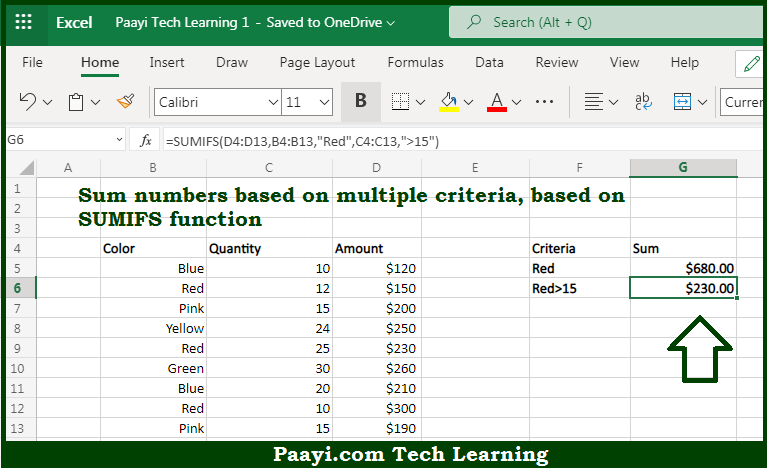# Learn How to SUM If Multiple Criteria in Microsoft Excel

Written by | 0 Comments | 525 Views

In this article, you will learn how to SUM various things in Microsoft Excel using a single/combination(s) of functions. You will also know how to SUM If Multiple Criteria and see the generic formula.

SUM If Multiple Criteria in Microsoft Excel

The main purpose of this formula is to sum numbers based on multiple criteria. Here we will learn how to sum if multiple criteria work in the workbook in Microsoft Excel. That implies, with the help of a formula based on the SUMIFS function you can able to sum numbers based on multiple criteria. So, with the help of this formula, you can able to sum if multiple criteria work in the workbook in Microsoft Excel.

General Formula to SUM If Multiple Criteria

=SUMIFS(sum_rng, crit_rng1, crit1, crit_rng2, crit2)

The Explanation for the SUM If Multiple CriteriaSo we know that with the help of the given formula above you can able to sum numbers based on multiple criteria. Here we will learn how to sum if multiple criteria work in the workbook in Microsoft Excel. As we know that the first range provided here is (D4:D11) are the cells to sum, called the "sum range". It should be noted that the criteria are supplied in pairs, that is range/criteria. So, with the help of this formula, you can able to sum if multiple criteria work in the workbook in Microsoft Excel.